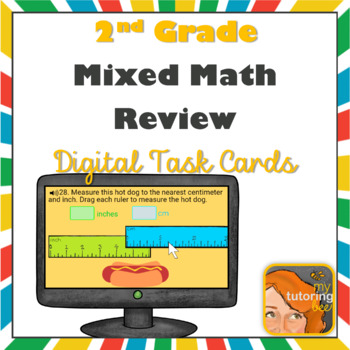2nd Grade math review assessment Boom Cards Distance Learning2nd - 3rd
Subjects
Standards
Resource Type
Formats Included
• Zip
• Internet Activities
Pages
30 pages
Compatible with Digital Devices
The Teacher-Author has indicated that this resource can be used for device-based learning.

Description

Your students will love this 2nd grade math review Boom Cards deck! I use this deck as an assessment for 2nd graders or as a review for 3rd graders at the beginning of the year. Perfect for back to school.

Students can work on this independently or teachers can use the included answer key to more accurately assess student knowledge.

If you have never tried digital task cards before, I include all of the instructions you'll need to get started!

You can preview the first 4 cards of this deck here!

• Boom Card Deck for 2nd grade
• video explaining how I use this resource

This deck includes a variety of question types including:

• Addition/Subtraction through the hundreds place
• Mental math
• Equal groups
• Arrays
• Place value awareness
• Standard, word and expanded form
• Comparing
• Place value alignment when adding/subtracting
• Calendar fluency
• Number patterns
• Multi-step word problems
• Various word problem operations
• Counting, adding and subtracting money
• Algebraic reasoning
• Measurement tools
• Estimating and finding lengths with standard units
• Number line
• Telling time
• Graphs
• 2-D shape attributes
• Equal parts in fractions
• Skip counting

If you like this set of digital task cards, you may also like:

Total Pages
30 pages
Included
Teaching Duration
N/A
Report this Resource to TpT
Reported resources will be reviewed by our team. Report this resource to let us know if this resource violates TpT’s content guidelines.

Standards

to see state-specific standards (only available in the US).
Partition circles and rectangles into two, three, or four equal shares, describe the shares using the words halves, thirds, half of, a third of, etc., and describe the whole as two halves, three thirds, four fourths. Recognize that equal shares of identical wholes need not have the same shape.
Recognize and draw shapes having specified attributes, such as a given number of angles or a given number of equal faces. Identify triangles, quadrilaterals, pentagons, hexagons, and cubes.
Use addition to find the total number of objects arranged in rectangular arrays with up to 5 rows and up to 5 columns; write an equation to express the total as a sum of equal addends.
Determine whether a group of objects (up to 20) has an odd or even number of members, e.g., by pairing objects or counting them by 2s; write an equation to express an even number as a sum of two equal addends.
Fluently add and subtract within 20 using mental strategies. By end of Grade 2, know from memory all sums of two one-digit numbers.